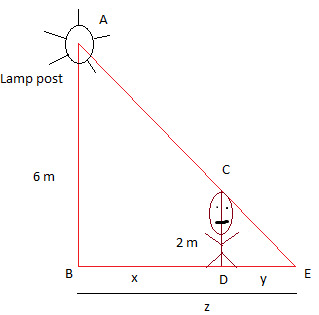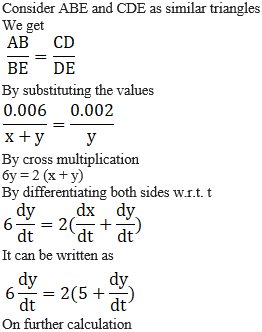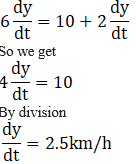# RS Aggarwal Solutions for Class 12 Maths Chapter 11: Applications of Derivatives Exercise 11A

Derivative as a rate measure is the topic which is covered under the Exercise 11A of Chapter 11. It also contains various solved examples, which can be referred by students to understand steps followed in answering them. Students can use the solutions PDF as a major study material to boost their exam preparation. Here the students can download RS Aggarwal Solutions Class 12 Maths Chapter 11 Applications of Derivatives Exercise 11A PDF from the links given below.

## RS Aggarwal Solutions for Class 12 Maths Chapter 11: Applications of Derivatives Exercise 11A Download PDF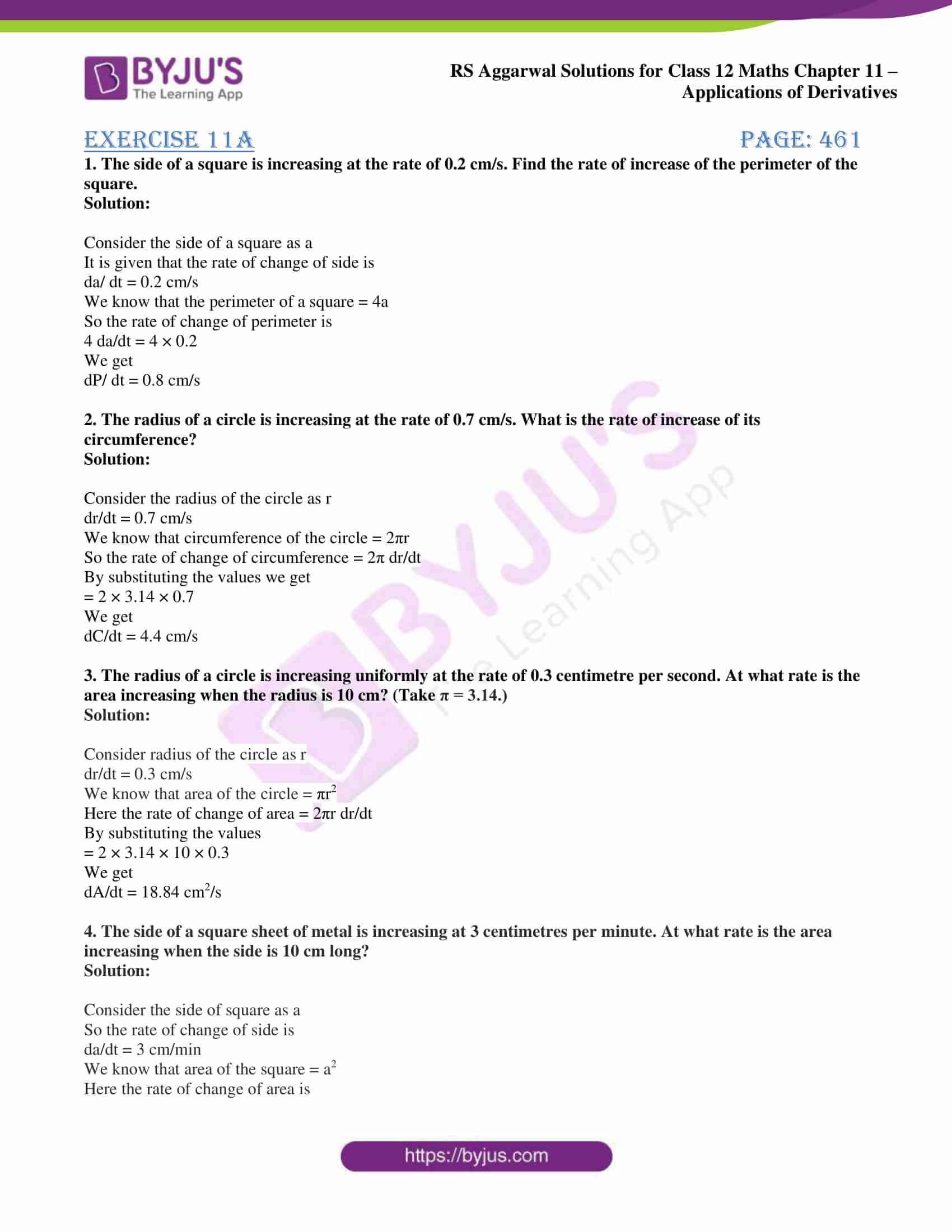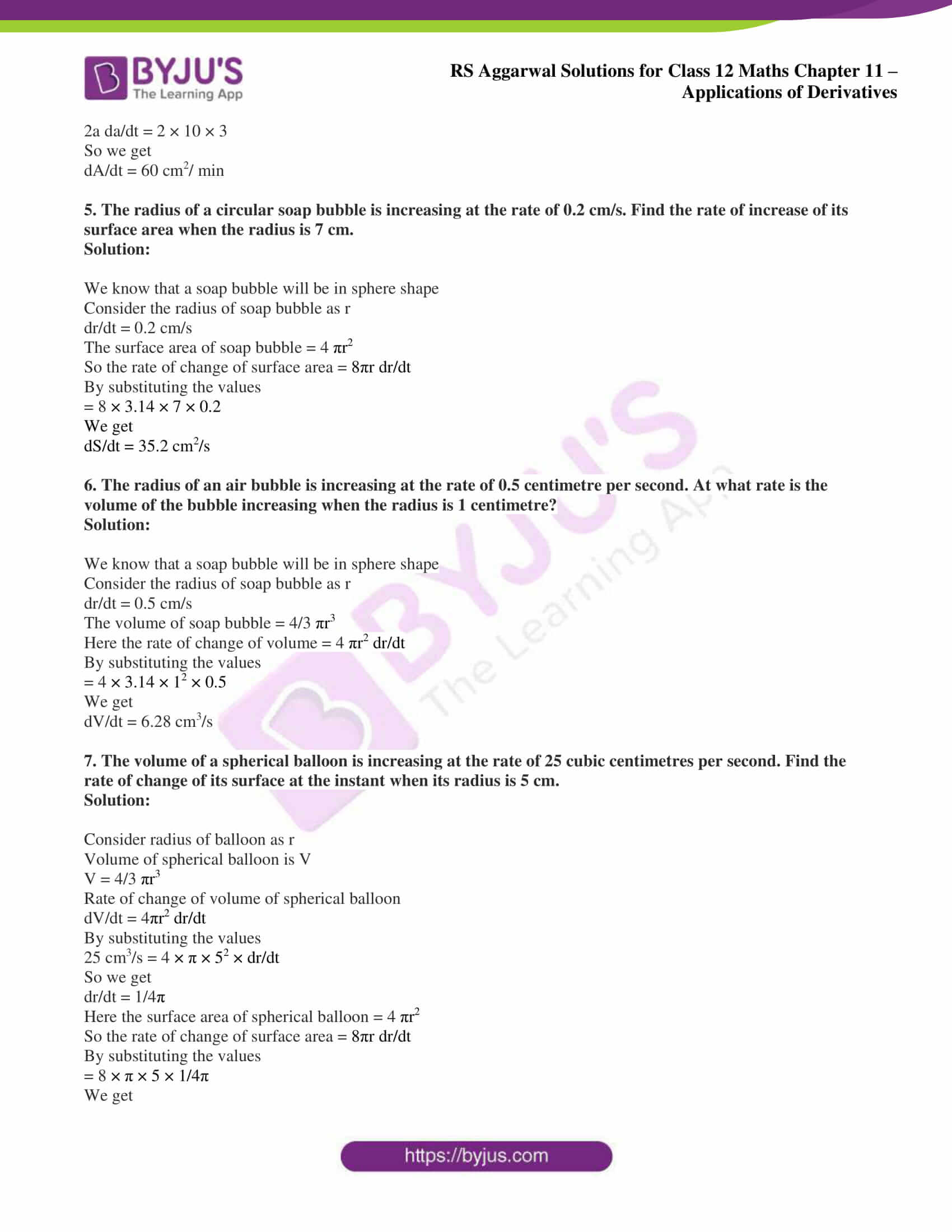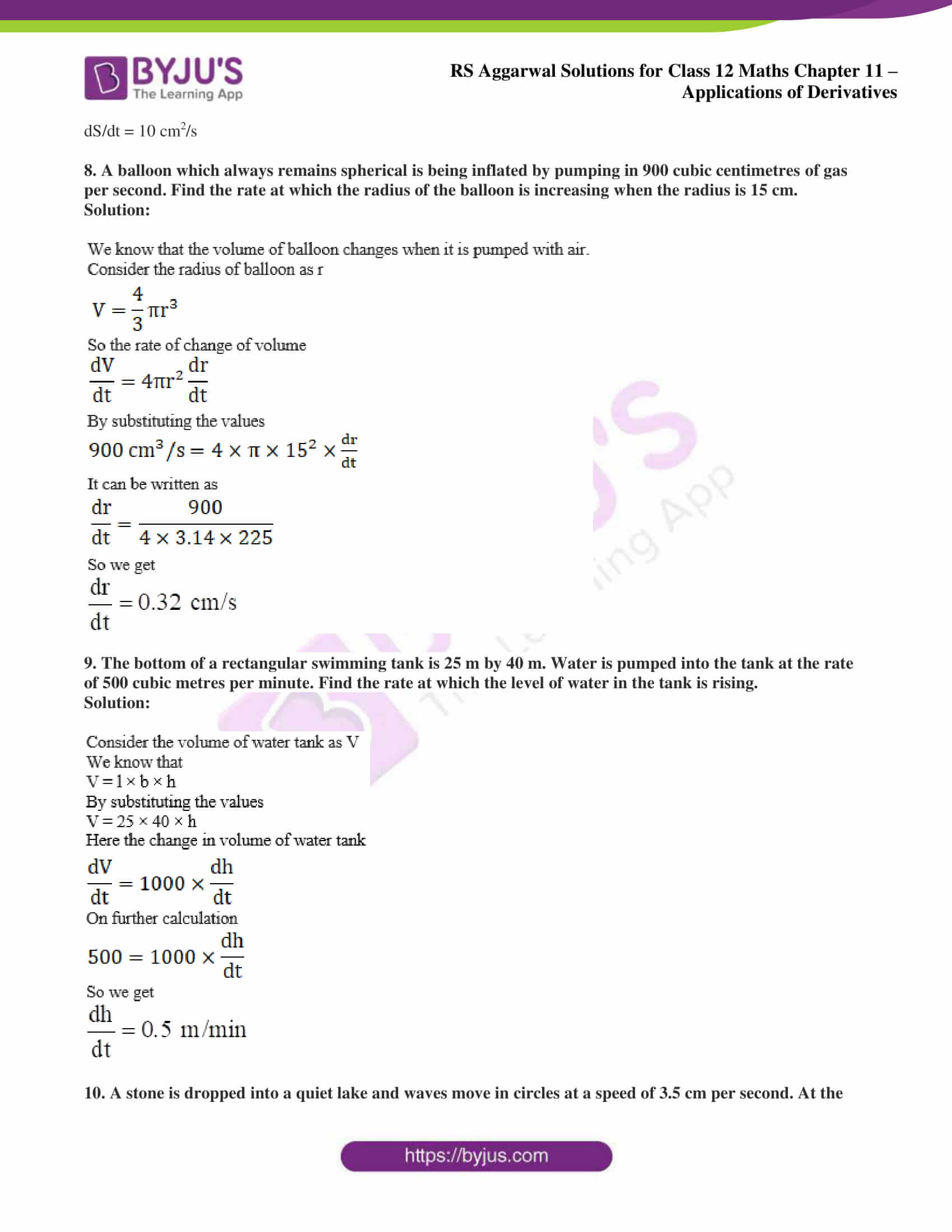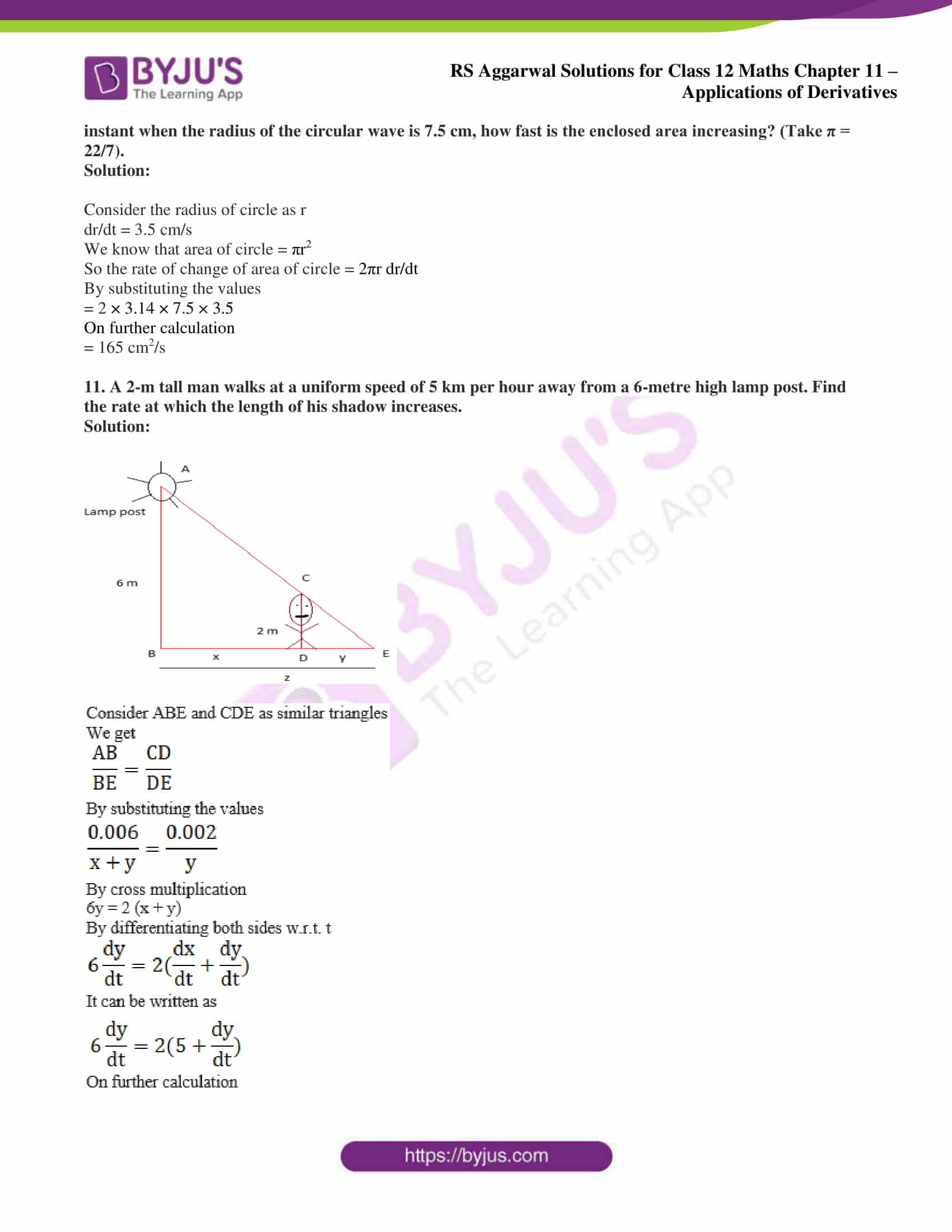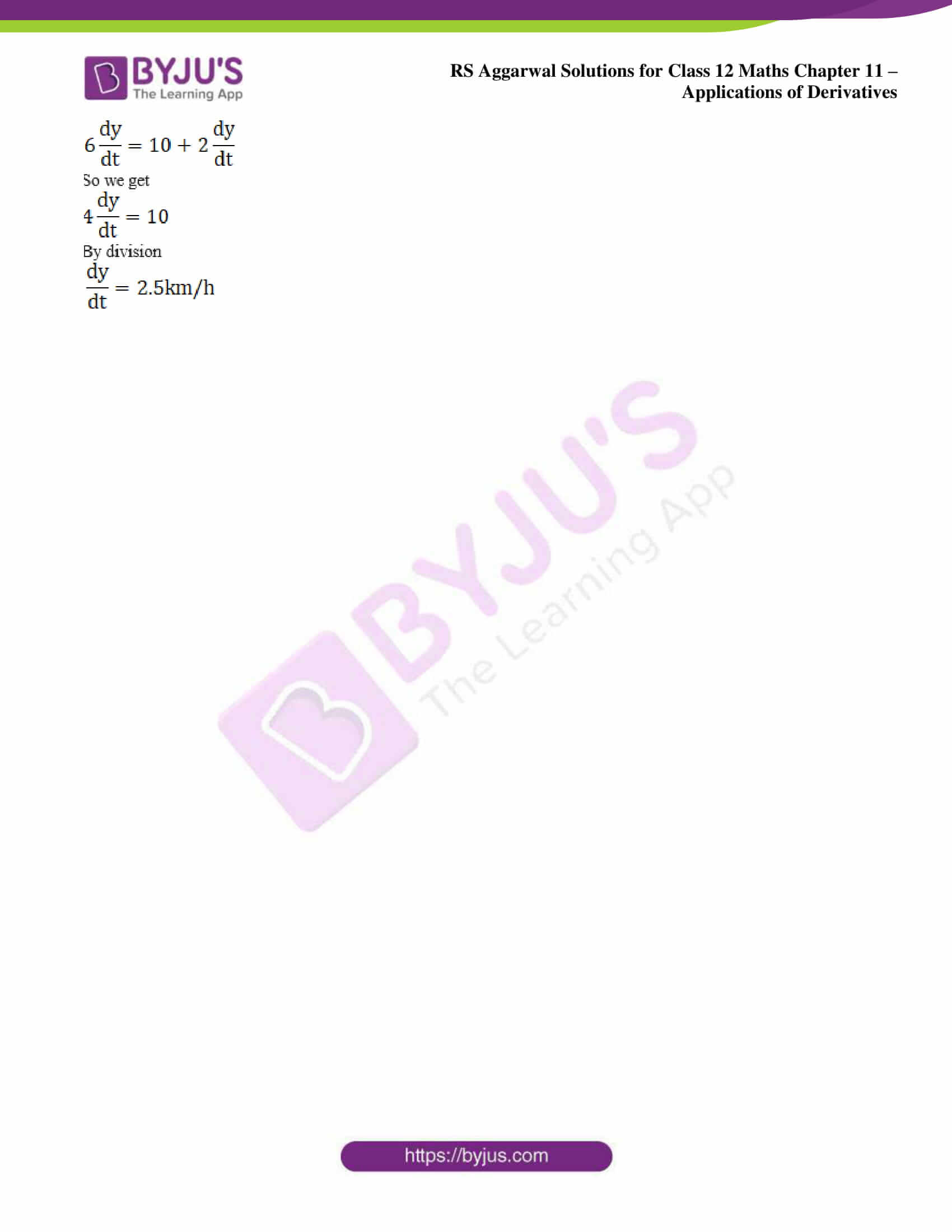### Access other exercise solutions of Class 12 Maths Chapter 11: Applications of Derivatives

Exercise 11B Solutions

Exercise 11C Solutions

Exercise 11D Solutions

Exercise 11E Solutions

Exercise 11F Solutions

Exercise 11G Solutions

Exercise 11H Solutions

### Access RS Aggarwal Solutions for Class 12 Maths Chapter 11: Applications of Derivatives Exercise 11A

1. The side of a square is increasing at the rate of 0.2 cm/s. Find the rate of increase of the perimeter of the square.

Solution:

Consider the side of a square as a

It is given that the rate of change of side is

da/ dt = 0.2 cm/s

We know that the perimeter of a square = 4a

So the rate of change of perimeter is

4 da/dt = 4 × 0.2

We get

dP/ dt = 0.8 cm/s

2. The radius of a circle is increasing at the rate of 0.7 cm/s. What is the rate of increase of its circumference?

Solution:

Consider the radius of the circle as r

dr/dt = 0.7 cm/s

We know that circumference of the circle = 2πr

So the rate of change of circumference = 2π dr/dt

By substituting the values we get

= 2 × 3.14 × 0.7

We get

dC/dt = 4.4 cm/s

3. The radius of a circle is increasing uniformly at the rate of 0.3 centimetre per second. At what rate is the area increasing when the radius is 10 cm? (Take π = 3.14.)

Solution:

Consider radius of the circle as r

dr/dt = 0.3 cm/s

We know that area of the circle = πr2

Here the rate of change of area = 2πr dr/dt

By substituting the values

= 2 × 3.14 × 10 × 0.3

We get

dA/dt = 18.84 cm2/s

4. The side of a square sheet of metal is increasing at 3 centimetres per minute. At what rate is the area increasing when the side is 10 cm long?

Solution:

Consider the side of square as a

So the rate of change of side is

da/dt = 3 cm/min

We know that area of the square = a2

Here the rate of change of area is

2a da/dt = 2 × 10 × 3

So we get

dA/dt = 60 cm2/ min

5. The radius of a circular soap bubble is increasing at the rate of 0.2 cm/s. Find the rate of increase of its surface area when the radius is 7 cm.

Solution:

We know that a soap bubble will be in sphere shape

Consider the radius of soap bubble as r

dr/dt = 0.2 cm/s

The surface area of soap bubble = 4 πr2

So the rate of change of surface area = 8πr dr/dt

By substituting the values

= 8 × 3.14 × 7 × 0.2

We get

dS/dt = 35.2 cm2/s

6. The radius of an air bubble is increasing at the rate of 0.5 centimetre per second. At what rate is the volume of the bubble increasing when the radius is 1 centimetre?

Solution:

We know that a soap bubble will be in sphere shape

Consider the radius of soap bubble as r

dr/dt = 0.5 cm/s

The volume of soap bubble = 4/3 πr3

Here the rate of change of volume = 4 πr2 dr/dt

By substituting the values

= 4 × 3.14 × 12 × 0.5

We get

dV/dt = 6.28 cm3/s

7. The volume of a spherical balloon is increasing at the rate of 25 cubic centimetres per second. Find the rate of change of its surface at the instant when its radius is 5 cm.

Solution:

Consider radius of balloon as r

Volume of spherical balloon is V

V = 4/3 πr3

Rate of change of volume of spherical balloon

dV/dt = 4πr2 dr/dt

By substituting the values

25 cm3/s = 4 × π × 52 × dr/dt

So we get

dr/dt = 1/4π

Here the surface area of spherical balloon = 4 πr2

So the rate of change of surface area = 8πr dr/dt

By substituting the values

= 8 × π × 5 × 1/4π

We get

dS/dt = 10 cm2/s

8. A balloon which always remains spherical is being inflated by pumping in 900 cubic centimetres of gas per second. Find the rate at which the radius of the balloon is increasing when the radius is 15 cm.

Solution: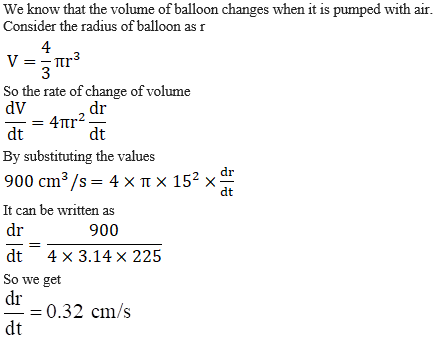9. The bottom of a rectangular swimming tank is 25 m by 40 m. Water is pumped into the tank at the rate of 500 cubic metres per minute. Find the rate at which the level of water in the tank is rising.

Solution: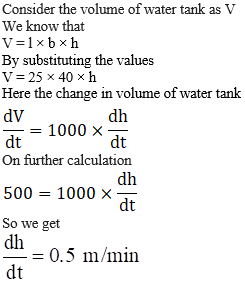10. A stone is dropped into a quiet lake and waves move in circles at a speed of 3.5 cm per second. At the instant when the radius of the circular wave is 7.5 cm, how fast is the enclosed area increasing? (Take π = 22/7).

Solution:

Consider the radius of circle as r

dr/dt = 3.5 cm/s

We know that area of circle = πr2

So the rate of change of area of circle = 2πr dr/dt

By substituting the values

= 2 × 3.14 × 7.5 × 3.5

On further calculation

= 165 cm2/s

11. A 2-m tall man walks at a uniform speed of 5 km per hour away from a 6-metre high lamp post. Find the rate at which the length of his shadow increases.

Solution: